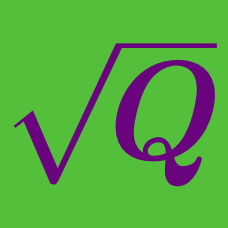Algebra

Radical Expressions and Equations: Level 4 Challenges

Determine the sum of all possible integer values of $x<100$ such that $y$ is an integer.

$\sqrt{x+\sqrt{x+\sqrt{x+\sqrt{x...}}}}=y$

If $\sqrt{x+3-4\sqrt{x-1}}+\sqrt{x+8-6\sqrt{x-1}}=1$ what is the sum of all integer values that $x$ can take?

$x-\sqrt{\frac{x}{2}+\frac{7}{8}-\sqrt{\frac{x}{8}+\frac{13}{64}}}=179$

Find the value of $10x$.

Inspiration

$\Large{\frac1{\sqrt{1}+\sqrt{2}+\sqrt{4}} + \frac1{\sqrt{4}+\sqrt{6}+\sqrt{9}} + \frac1{\sqrt{9}+\sqrt{12}+\sqrt{16}}}$

If the expression above can be simplified to the form of ${\sqrt a + b}$ for integers $a$ and $b$, find the value of $a+b$.

Source: $MA\Theta$ 1992.

The minimum value of $\sqrt{x^4 - x^2 - 24x + 145} + \sqrt{x^4 - 23x^2 - 2x + 145}$ can be expressed in the form $a\sqrt{b}$ , where $a$ and $b$ are integers, with $b$ is not divisible by the square of any prime. What is the value of $a+b$ ?

×